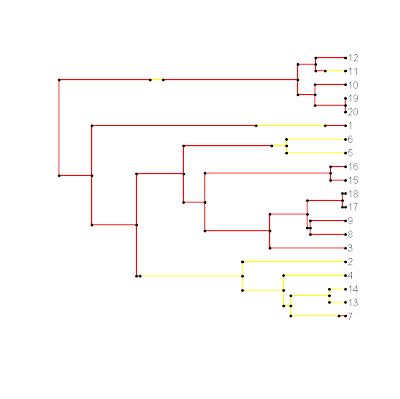## Tuesday, June 28, 2011

### Plot stochastic character mapped tree

I just completed a beta version of my plotSimmap() function. I'll post a little more about how this was accomplished tomorrow, but it was fairly straightforward after figuring out how to plot rooted phylograms generally (see previous post here). To check it out, download it (source here), load the source into R, and then run the following code (for example):

> require(geiger)
> require(phytools)
> tree<-drop.tip(birthdeath.tree(b=1,d=0,taxa.stop=21),"21")
> x<-sim.char(tree,model.matrix=list(matrix(c(-1,1,1,-1),2,2)),
model="discrete")[,,1]
> mtree<-make.simmap(tree,x)
> legend<-c("red","yellow"); names(legend)<-c(1,2)
> source("plotSimmap.R")
> plotSimmap(mtree,legend)